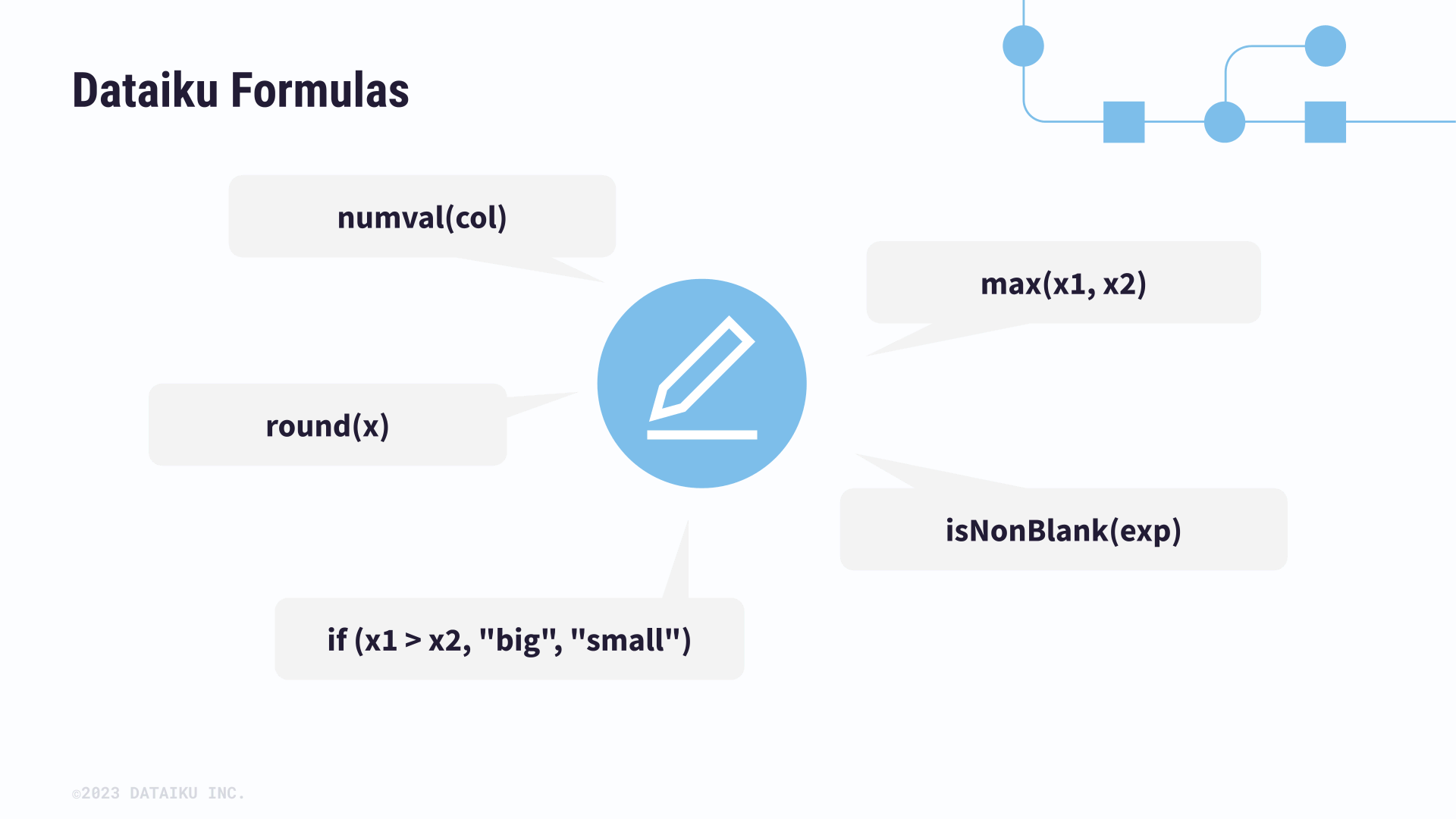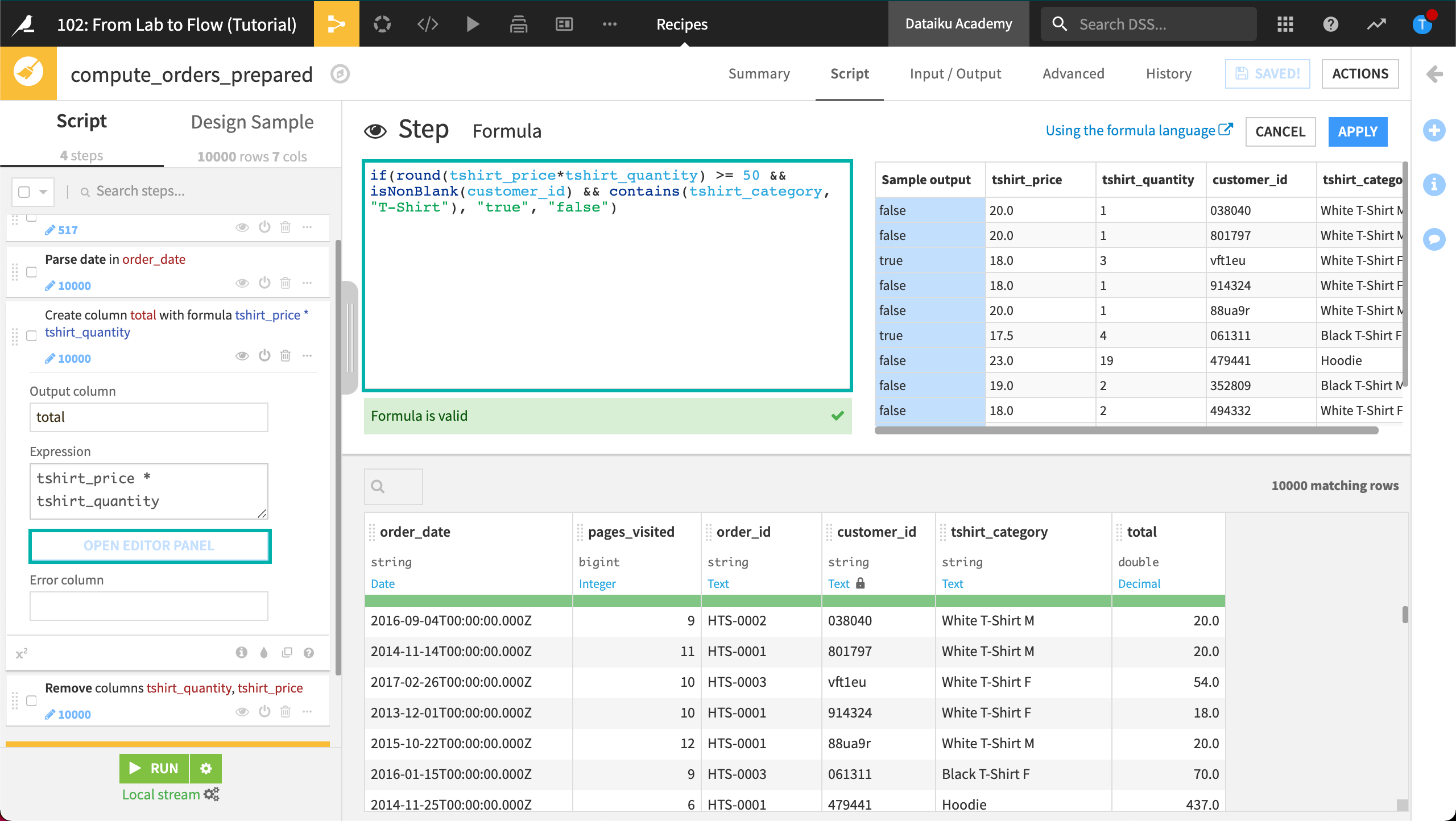# Concept: Formulas in DSS¶

Often in a Prepare recipe, you will want to create new columns based on those already present in your dataset. In the world of machine learning, this is called feature generation.

Similar to what you might find in a spreadsheet tool like Excel, DSS has its own Formula language.

It is a powerful expression language to perform calculations, manipulate strings, and much more.From the processor library, you can add a Formula step and provide the name of the output column.

You could write simple formulas directly in the Expression box. Clicking the Edit button, however, adds a few support measures. The first is code completion. As soon as you start typing, DSS starts suggesting columns from the dataset or functions to apply. The Editor will also alert you if the formula is invalid.

The Formula language allows you to craft expressions of considerable complexity. For example, you can use:

• common mathematical functions, such as round, sum and max

• comparison operators, such as >, <, >=, <=

• logical operators, such as AND and OR

• tests for missing values, such as isBlank() or isNULL()

• string operations with functions like contains(), length(), and startsWith()

• conditional if-then statements